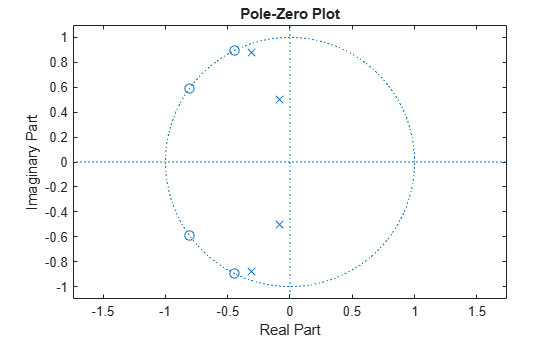# zplane

Z-plane zero-pole plot for discrete-time filter System object

## Syntax

```zplane(filt) zplane(filt,Name,Value) [zLoc,pLoc,tLoc] = zplane(filt) ```

## Description

`zplane(filt)` plots the zeros and poles of the filter System object™, `filt`, with the unit circle for reference in the filter visualization tool (fvtool). Each zero is represented with a `'o'` and each pole with a `'x'` on the plot. Multiple zeros and poles are indicated by the multiplicity number shown to the upper right of the zero or pole.

When you call the `step` method on the filter System object with a fixed-point input, the filter becomes a quantized fixed-point filter, `filtQuant`. When `filtQuant` is a quantized filter, `zplane(filtQuant)` plots the poles and zeros of the quantized and unquantized filters. The symbolsand `+` represent the zeros and poles of the quantized filter `filtQuant`. The plot includes the unit circle for reference.

`zplane(filt,Name,Value)` returns the pole zero plot with additional options specified by one or more `Name,Value` pair arguments.

```[zLoc,pLoc,tLoc] = zplane(filt)``` returns the vector of locations for zeros, poles, and text objects. `zLoc` is the vector of locations of zeros, `pLoc` is the vector of locations of poles, and `tLoc` is the vector of locations of the axes/unit circle line and text objects which are present when there are multiple zeros or poles. In case there are no zeros or poles, `zLoc` or `pLoc` is set to the empty matrix `[]`.

## Input Arguments

collapse all

 `filt` A filter System object. The following Filter System objects are supported by this analysis function:

### Name-Value Pair Arguments

Specify optional comma-separated pairs of `Name,Value` arguments. `Name` is the argument name and `Value` is the corresponding value. `Name` must appear inside quotes. You can specify several name and value pair arguments in any order as `Name1,Value1,...,NameN,ValueN`.

When you specify `'double'` or `'single'`, the function performs double- or single-precision analysis. When you specify `'fixed'` , the arithmetic changes depending on the setting of the `CoefficientDataType` property and whether the System object is locked or unlocked.

When you do not specify the arithmetic for non-CIC structures, the function uses double-precision arithmetic if the filter System object is in an unlocked state. If the System object is locked, the function performs analysis based on the locked input data type. CIC structures only support fixed-point arithmetic.

## Output Arguments

 `zLoc` Vector of locations of zeros. `pLoc` Vector of locations of poles. `tLoc` Vector of locations of axes/unit circle line and text objects.

## Examples

collapse all

Create a Fourth-order IIR digital filter with a cutoff frequency of 0.6. Plot the poles and zeros of this filter.

```[b,a] = ellip(4,.5,20,.6); zplane(b,a);```Quantize the filter by passing a fixed-point input through the filter algorithm. Plot the quantized and unquantized poles and zeros associated with this filter.

```iirFilt = dsp.IIRFilter('Numerator',b,'Denominator',a); in = fi(randn(15,6),1,15,3); out = iirFilt(in); zplane(iirFilt)```